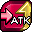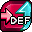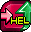13,610 Pages

Parameter ConversionBuff ID 24 40

Parameter Conversion is an effect that uses a specified portion of one stat and adds it to another stat. It is a flat bonus added after total stat calculations. The bonus is not affected by multipliers to the stat it is being added to, but is affected by multipliers to the stat that is being converted from.

If multiple forms of stacking conversion effects exist, each effect is applied individually; however, all conversion buffs from the same category to a single stat will not stack with each other. For example, a Def to Atk conversion buff from BB/SBB cannot stack with a Rec to Atk conversion buff from BB/SBB, but a Def to Atk conversion buff from BB/SBB can stack with a Rec to Atk conversion buff from UBB can and vice-versa.

Parameter Conversion buffs ignore the bonus to a stat from other stat conversions.

## Formula

```Total Atk = Base Atk * (Total Buff Atk + Total Passive Atk) + Atk Conversion Buff

Total Def = Base Def * (Total Buff Def + Total Passive Def) + Def Conversion Buff

Total Rec = Base Rec * (Total Buff Rec + Total Passive Rec) + Rec Conversion Buff
```

## Example

An OE+0 max-leveled and max-imped Ancient Calamity Arumat has the "100% boost to Atk, max HP" unlocked with no other parameter boosts from Leader Skill available. Arumat should have the following:

• 34000 HP, 11600 Atk, 2000 Def, 1400 Rec
• An 80% Def to Atk conversion buff would result in Arumat's Atk stat being 13200
• Note that any other conversion buff that converts to Atk will overwrite this buff